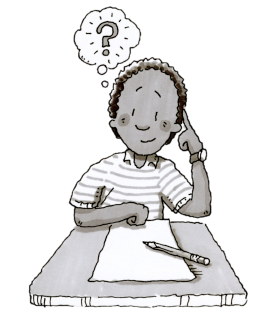Home > CC2MN > Chapter cc23 > Lesson cc23.2.3 > Problem3-63

3-63.Nathan wants you to solve this puzzle: “I am thinking of a number. If you divide my number by $\mathit{3}$ and add $\mathit{-3}$, you will get $\mathit{4}$. What is my number?” Show all of your work. Homework Help ✎

 Hint:Work backwards to get Nathan's number.Hint 2:Start with the end number, which is $4$.Hint 3:Now instead of adding $-3$, subtract $-3$.You should now have $7$.More Help:Instead of dividing $7$ by $3$, multiply it by $3$ to get Nathan's number.